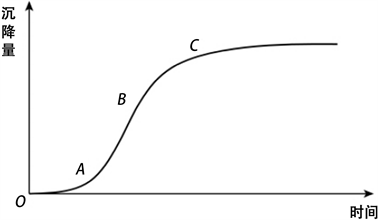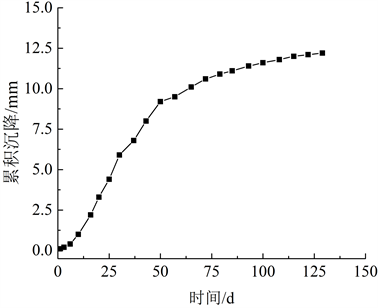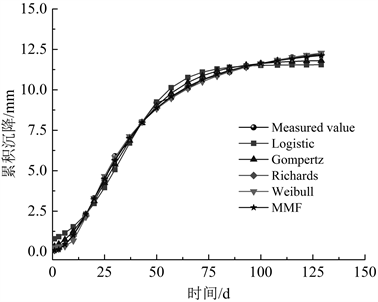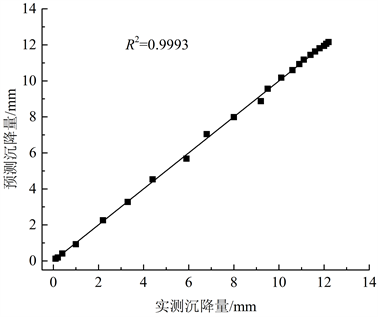#### 期刊菜单

Analysis of Surface Settlement Prediction of Tunnel Portal Section Construction Based on the S-Shaped Composite Curves
DOI: 10.12677/HJCE.2022.114068, PDF , HTML, XML, 下载: 132  浏览: 184  科研立项经费支持

Abstract: The construction of tunnel portal section is easy to cause a large area of settlement on the overlying surface, which poses a great threat to the stability of tunnel entrance slope. The surface accumulated settlement caused by construction usually shows an S-shaped variation law. This paper selects five commonly used S-shape curves (Logistic, Gompertz, Richards, Weibull and MMF curves) and respectively applies them to the prediction of ground surface settlement caused by Huangnigangtao tunnel, and the prediction effects of each single model are analyzed. The weighted average algorithm is used to obtain the combined weight values of five curves and the S-shaped composite curve is built. Results show that MMF has the best fitting effect among five single S-shaped curves. The combined model was obtained by weight optimization, and its fitting evaluation R2 and RMSE values were better than that of the single models, which improved the prediction accuracy of surface subsidence and verified the validity of the proposed method.

1. 引言

Logistic、Gompertz、Richards、Weibull及MMF等5种S型曲线均能在一定程度上反映地表沉降发展规律，但是由于自身拟合特征的局限，难以适应不同工况条件的地表沉降预测。在考虑各曲线特征和局限性的基础上，运用加权平均算法，获得各种S型曲线的组合权重值，构建组合预测模型，并将其运用于浙江文成县黄泥岗头隧道洞口施工诱发地表沉降预测，验证组合预测模型的合理性。

2. 地表沉降规律Figure 1. Laws of ground surface settlement

① 初始沉降阶段OA：沉降速度较慢，沉降量随时间增加缓慢。

② 加速沉降阶段AB：沉降速度随时间增加而增大，并达到极限。也就是说，沉降呈快速增长趋势。

③ 稳定沉降阶段BC：随着时间的推移，沉降量继续增大，沉降速度逐渐减小。

④ 最终沉降阶段CD：沉降速度继续减小，最终趋于0。同时，沉降增长缓慢，接近极限。

3. 组合S型曲线预测模型

3.1. S型曲线

S型曲线又称增长曲线，被广泛运用于社会、经济预测，用于描述事情产生、发展、成熟最终达到极限状态的系统过程，比简单的时间序列技术更为准确 。S型曲线有多种形式，其中Logistic、Gompertz、Richards、Weibull及MMF曲线是较为常用的5种类型，均能较好地反映地表沉降发展过程。各曲线模型公式如下所示：

① Logistic曲线：

${y}_{1}\left(t\right)=\frac{a}{1+b{e}^{-ct}}$ (1)

② Gompertz曲线：

${y}_{2}\left(t\right)=a{e}^{-b{e}^{-ct}}$ (2)

③ Richards曲线：

${y}_{3}\left(t\right)=\frac{a}{1+{e}^{{\left(b-ct\right)}^{1/r}}}$ (3)

④ Weibull曲线：

${y}_{4}\left(t\right)=a-b{e}^{-c{t}^{r}}$ (4)

⑤ MMF曲线：

${y}_{5}\left(t\right)=\frac{bc+a{t}^{r}}{c+{t}^{r}}$ (5)

3.2. 组合S型曲线

$\underset{i=1}{\overset{m}{\sum }}{\omega }_{i}=1\text{\hspace{0.17em}}\text{\hspace{0.17em}}\text{\hspace{0.17em}}\left({\omega }_{i}\ge 0\right)$ (6)

${{x}^{\prime }}_{t}=\underset{i=1}{\overset{m}{\sum }}{\omega }_{i}{x}_{it}$ (7)

${e}_{t}^{i}={x}_{t}-{x}_{t}^{i}$ 为t时刻第i种预测方法的误差，则该时刻组合模型的误差为：

${e}_{t}={x}_{t}-{{x}^{\prime }}_{t}=\underset{i=1}{\overset{m}{\sum }}{\omega }_{i}\left({x}_{t}-{x}_{it}\right)=\underset{i=1}{\overset{m}{\sum }}{\omega }_{i}{e}_{it}$ (8)

$E=\underset{t=1}{\overset{N}{\sum }}{e}_{t}^{2}=\underset{t=1}{\overset{N}{\sum }}{\left(\underset{i=1}{\overset{m}{\sum }}{\omega }_{i}{e}_{it}\right)}^{2}$ (9)

$\left\{\begin{array}{c}\mathrm{min}E\\ \underset{i=1}{\overset{m}{\sum }}{\omega }_{i}=1\\ {\omega }_{i}\ge 0\end{array}$ (10)

4. 工程应用

4.1. 工程概况

4.2. 单一曲线预测Figure 2. Measured cumulative surface settlementFigure 3. Comparison of predicted and measured curvesTable 1. Fitting parameters of S-shaped curves

4.3. 组合预测分析Table 2. Combination weights of S-shaped curvesFigure 4. Comparison of predicted and measured settlement

4.4. 对比分析

$RMSE=\sqrt{\frac{1}{N}\underset{i=1}{\overset{N}{\sum }}{\left(y-{y}^{\prime }\right)}^{2}}$ (11)Table 3. Values of R2 and RMSE for each model

5. 结论

1) 根据地表沉降的发展规律和对现场监测数据的分析，地表沉降的发展过程可分为初始沉降阶段、加速沉降阶段、稳定沉降阶段和最终沉降阶段四个阶段。隧道洞口施工导致地表沉降规律与S型曲线的变化规律一致。

2) 将5种S型曲线应用于浙江文成县黄泥岗头隧道洞口诱发地表沉降预测，均取得了较好的效果。在单一预测模型中，MMF模型预测精度最高，说明其曲线特性更加适合于隧道洞口段施工地表沉降规律。

3) 基于加权平均算法的组合预测模型弥补了单一预测模型对地表沉降预测的不足，充分发挥各曲线适合不同工况的特征，减小不利特征对沉降预测的影响，有效地提高了隧道洞口段施工地表沉降预测精度。

  宋卫东, 谢政平, 张继清. 天坛东门站浅埋暗挖施工顺序对地表沉降影响的数值模拟分析[J]. 岩石力学与工程学报, 2005, 24(S2): 5773-5778.  董俊. 地铁过街通道矩形顶管施工变形监测分析[J]. 铁道工程学报, 2016, 33(8): 106-110.  张文龙. 郑州地铁浅埋暗挖通道施工引起的沉降分析[J]. 河南理工大学学报(自然科学版), 2017, 36(5): 127-132.  李荣伟, 潘兴军. 砂土地基顶管稳定性分析及施工技术[J]. 三峡大学学报(自然科学版), 2019, 41(S1): 29-32.  吴锋波, 郑卫强, 齐剑峰, 等. 地铁双线盾构区间地表横向沉降槽参数分析[J]. 地下空间与工程学报, 2021, 17(5): 1653-1663.  Chen, C.-H., Wu, L. and Peng, Y.-X. (2016) The Analysis Method of Rock Mass Deformation of Large Cross-Section Tunnel. Geotechnical and Geological Engineering, 34, 739-743. https://doi.org/10.1007/s10706-015-9974-5  张仪萍. 地基沉降泊松曲线拟合的概率方法[J]. 岩土工程学报, 2005, 27(7): 837-840.  倪贤, 徐颖, 王峰, 等. 基于S型曲线的爆破挤淤工后沉降预测[J]. 工程爆破, 2020, 26(6): 50-55.  梅国雄, 宰金珉, 殷宗泽, 等. 沉降-时间曲线呈“S”型的证明及其应用——从土体本构关系[J]. 岩土力学, 2005, 26(s1): 21-24.  赵明华, 刘煜, 曹文贵. 软土路基沉降变权重组合S型曲线预测方法研究[J]. 岩土力学, 2005, 26(9): 1443-1447.  朱珉仁. Morgan-Mercer-Flodin模型和Weibull模型的拟合[J]. 数学的实践与认识, 2003, 33(1): 1-4.